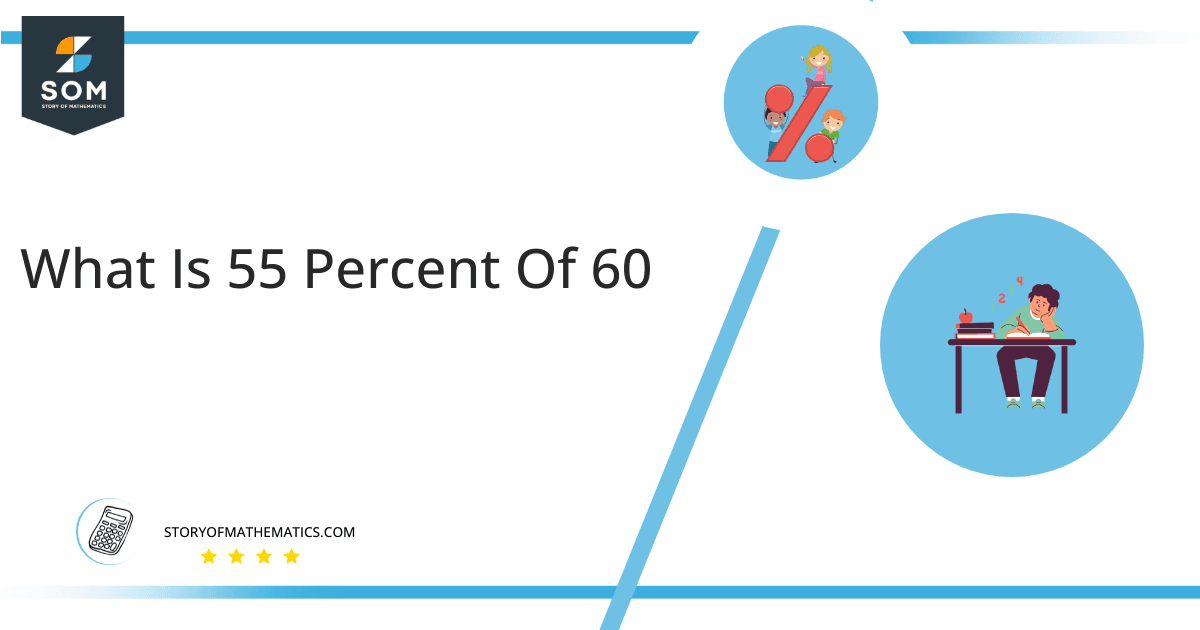# What is 55 percent of 60 + Solution With Free Steps55 percent of 60 is equal to 33. To get 33 as your answer, simply multiply the fraction 0.55 by the number 60.

The simple calculation of 55 percent of 60 can sum up as a real-life example, suppose, you wish to rent a room for 60 dollars a month. But the dealer says that you have to pay a security of 55% of that amount right now, now if you already know that 55 percent of 60 is equal to 33, you can easily pay the amount without waiting to calculate it. Based on this assumption we can easily cater to such scenarios which require proportion and ratios.

## What Is 55 percent of 60?

The 55 percent of 60 is equal to the number 33. The multiplication result of the fraction 0.55 and the number 60 will produce the numeral 33.

To produce the answer quickly, take the fraction 55/100 and multiply it by 60, the result will produce 33 upon solving the equation.

## How To Calculate 55% of 60?

The below steps are carried out to calculate the percent value of the given amount:## Step 1

Firstly, translating 55 percent of 60 in terms of multiplication as:

55 percent of 60 = 55% x 60

## Step 2

Inserting 1/100 in place of percentage sign %:

Thus, Utilize the above equation.

55 percent of 60 = (55/100) x 60

## Step 3

Rearranging the above equation gives:

55 percent of 60 = (55 x 60) / 100

## Step 4

Eliminating 10 from the above equation and simplifying:

55 percent of 60 = (55 x 6) / 10

## Step 5

Multiplying 55 by 6 and then dividing it by 10 gives:

55 percent of 60 = 33

Therefore 55 percent of 60 is equal to 33.

The following pie chart displays 55 percent of 60.In the above Pie chart, the orange portion displays 55% of 60. The whole circle comprises 60 parts, of which 55 percent has to be determined. The calculation shows that it is composed of 33 portions. The green portion in the figure portrays the remaining part of 60 which is 45% equal to 27 as 60 – 33 = 27.

All the Mathematical drawings/images are created using GeoGebra.